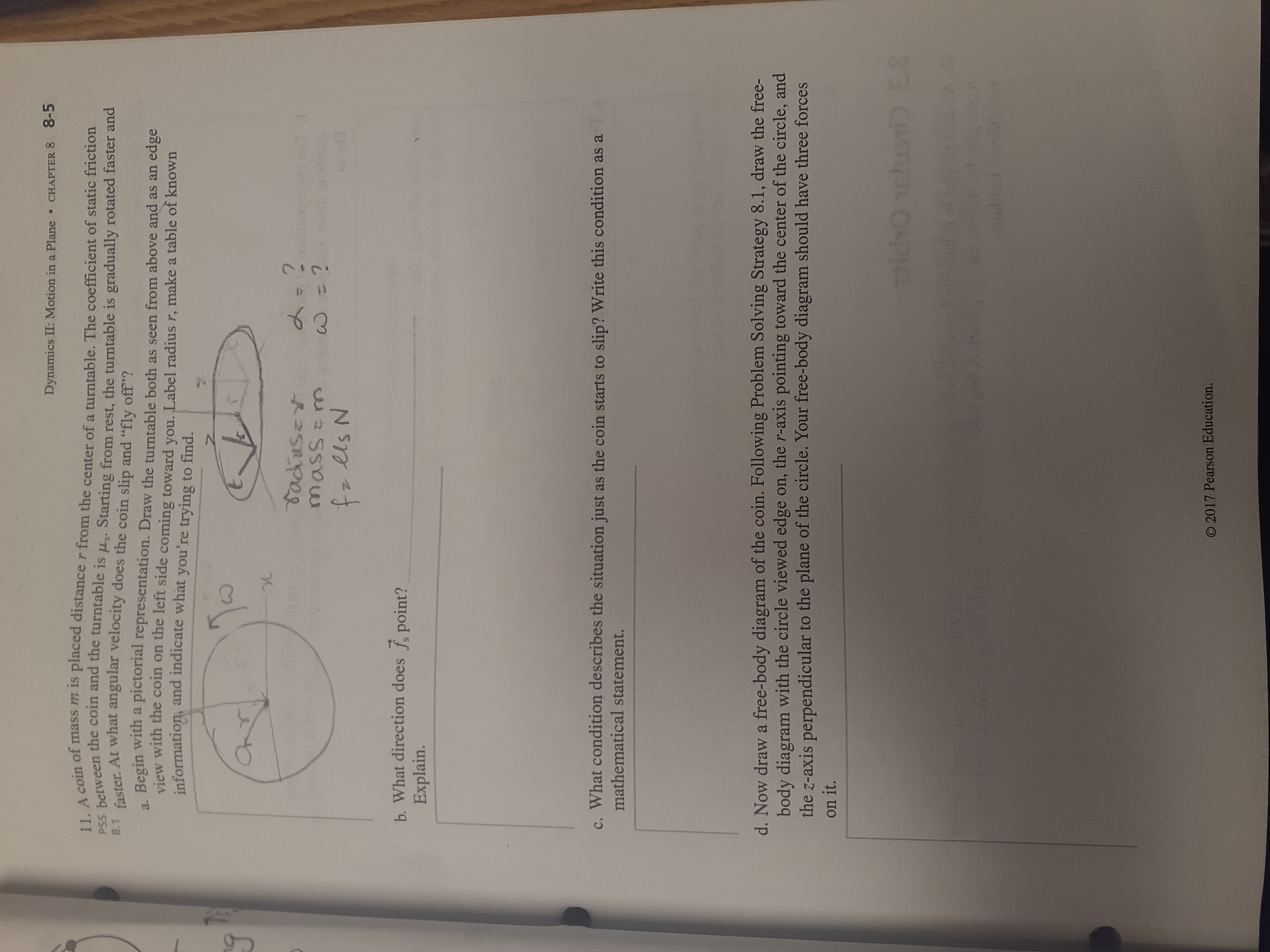# Dynamics II: Motion in a Plane8-5CHAPTER 811. A coin of mass m is placed distance r from the center of a turntable. The coefficient of static frictionPSS between the coin and the turntable is us. Starting from rest, the turntable is gradually rotated faster andfaster. At what angular velocity does the coin slip and "fly off"?a. Begin with a pictorial representation. Draw the turntable both as seen from above and as an edge8.1view with the coin on the left side coming toward you. Label radius r, make a table of knowninformation, and indicate what you're trying to find.OnTTadiusetMass z mfz els Nb. What direction does f point?Explain.c. What condition describes the situation just as the coin starts to slip? Write this condition as amathematical statement.d. Now draw a free-body diagram of the coin. Following Problem Solving Strategy 8.1, draw the free-body diagram with the circle viewed edge on, the r-axis pointing toward the center of the circle, andthe z-axis perpendicular to the plane of the circle. Your free-body diagram should have three forceson it.1tidOichoni E3O 2017 Pearson Education.D 13

Questionhelp_outlineImage TranscriptioncloseDynamics II: Motion in a Plane 8-5 CHAPTER 8 11. A coin of mass m is placed distance r from the center of a turntable. The coefficient of static friction PSS between the coin and the turntable is us. Starting from rest, the turntable is gradually rotated faster and faster. At what angular velocity does the coin slip and "fly off"? a. Begin with a pictorial representation. Draw the turntable both as seen from above and as an edge 8.1 view with the coin on the left side coming toward you. Label radius r, make a table of known information, and indicate what you're trying to find. OnT Tadiuset Mass z m fz els N b. What direction does f point? Explain. c. What condition describes the situation just as the coin starts to slip? Write this condition as a mathematical statement. d. Now draw a free-body diagram of the coin. Following Problem Solving Strategy 8.1, draw the free- body diagram with the circle viewed edge on, the r-axis pointing toward the center of the circle, and the z-axis perpendicular to the plane of the circle. Your free-body diagram should have three forces on it. 1tidOichoni E3 O 2017 Pearson Education. D 13 fullscreen
check_circleExpert Solution
Step 1

Hey, since there are multiple subparts questions posted, we will answer first three questions. If you want any specific question to be answered then please submit that question only or specify the question number in your message.

Step 2

(a) The pictorial representation is

Step 3

(b) The static frictional force acts radially inwards to the cente...

### Want to see the full answer?

See Solution

#### Want to see this answer and more?

Solutions are written by subject experts who are available 24/7. Questions are typically answered within 1 hour*

See Solution
*Response times may vary by subject and question
Tagged in

### Angular Motion